Next: 2.2.2 Feature-Scale Transport Up: 2.2 Transport Kinetics Previous: 2.2 Transport Kinetics

## 2.2.1 Reactor-Scale Transport

Although process simulation in the reactor-scale region is not the scope of this work, some basic considerations of different transport models and typical characteristics of flux distribution on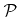are given in this section. The transport of particles can be characterized by the mean free path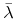. For an ideal gascalculates as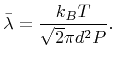(2.4)

Here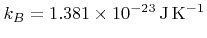denotes the Boltzmann constant,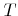is the temperature,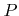is the pressure, and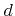is the collision diameter of a gas molecule, which is about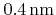for most molecules of interest .

If the mean free path is much smaller than the representative physical length scale, which is true in the case of the reactor dimension, the transport is said to be in the continuum regime [32,80]. This is usually the case for CVD and dry etching processes with typical pressures in the range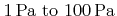and a typical temperature of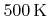resulting in a mean free path in the range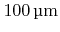to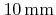. Hence, the velocities of a neutral particle species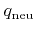can be assumed to follow the Maxwell-Boltzmann distribution, leading to a cosine-like directional dependence of the flux distribution on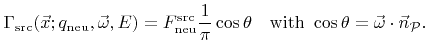(2.5)

Here the energy distribution is neglected, because the kinetic energy for typical process temperatures is usually too small to play a role in the surface reaction.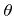is the angle between the incident direction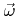and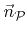.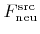is the flux onand can be assumed constant for all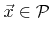at feature-scale. The value forcan either be obtained by calculating the reactor-scale transport or experimentally by measurements.

In many processes plasmas are used, leading to the need to model ion transport. Ions are accelerated towards the wafer surface due to the plasma sheath potential. This leads to a narrow angle distribution often described as power cosine distribution , also known as Phong distribution in the field of computer graphics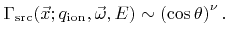(2.6)

For large exponents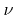this is equivalent to a normal distribution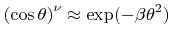with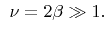(2.7)

Together with the energy distribution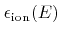where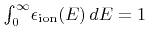the arrival flux distribution can be expressed as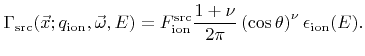(2.8)

If no collisions occur in the sheath, and if the final energy of ions is much larger than the initial random thermal energy,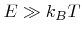, the cosine exponentcan be estimated by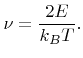(2.9)

For direct current (DC) biased plasmas the final ion energy can be assumed to be monoenergetic with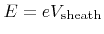, where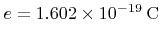denotes the elementary charge and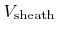is the sheath potential. Hence, the energy distribution can be approximated by a Dirac delta function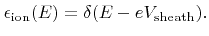(2.10)

Radio frequency (RF) biased plasmas result in more complex energy distributions with a characteristic bimodal shape. Plasma sheath simulations using MC techniques are able to predict the energy distribution function and can also incorporate ion collisions in the plasma sheath region [27,56].

For most processes the flux distribution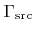does not vary significantly overat feature-scale. However, this is not true for processes working with local particle bombardments such as FIBs. The spatial intensity distribution of such a beam is usually described by a Gaussian profile [57,132,133]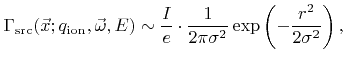(2.11)

where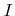denotes the beam current with typical values in the range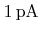toand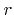is the distance from the center line of the beam.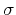is proportional to the beam diameter, which is commonly defined as the full width at half maximum (FWHM), thus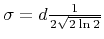.

At feature-scale it is a reasonable approximation to regard the beam as monoenergetic and unidirectional. If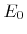is the ion beam energy, typically ranging from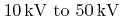, and if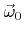represents the incidence direction, the arrival angular energy flux distribution can be written as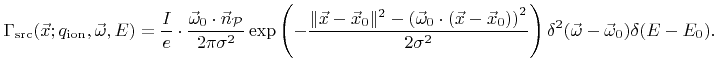(2.12)

Here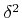denotes a two-dimensional delta distribution satisfying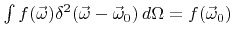.Next: 2.2.2 Feature-Scale Transport Up: 2.2 Transport Kinetics Previous: 2.2 Transport Kinetics

Otmar Ertl: Numerical Methods for Topography Simulation